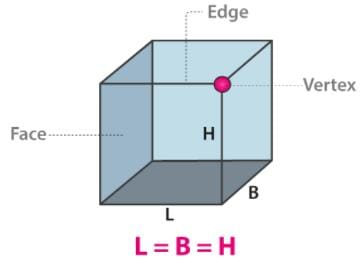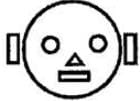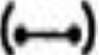# Test: Geometry - 1

## 10 Questions MCQ Test Mathematics for Class 3 | Test: Geometry - 1

Description
Attempt Test: Geometry - 1 | 10 questions in 15 minutes | Mock test for Class 3 preparation | Free important questions MCQ to study Mathematics for Class 3 for Class 3 Exam | Download free PDF with solutions
QUESTION: 1

### Which one has curved edge?

Solution:

Ring has curved edge.

QUESTION: 2

### Which one is a closed shape?

Solution:

Star a closed shape.

QUESTION: 3

### Mark the correct statement.

Solution:

Triangle has three sides.

QUESTION: 4

Which one is the 3-D shape in given shapes?

Solution:

Cuboids is a 3-D shape.

QUESTION: 5

A square has

Solution:

A square has four sides four corners.

QUESTION: 6

It has six flat faces and twelve straight edges

Solution:

A Cube is a solid three-dimensional figure, which has 6 square faces, 8 vertices and 12 edges. It is also said to be a regular hexahedron.QUESTION: 7This figure contains

Solution:

The figure is having 3 circles, 3 rectangles and 1 triangle.

QUESTION: 8

Which one of the following is different from the other three?

Solution:

Circle is different because it does not has sides.

QUESTION: 9

Which one of the following has six square faces?

Solution:

Cube has six square faces.

QUESTION: 10

How many units of cube are there in this figure?Solution:

6 units of cube are there in this figure.Use Code STAYHOME200 and get INR 200 additional OFF Use Coupon Code